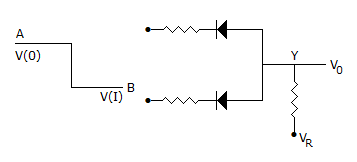# Electronics and Communication Engineering - Digital Electronics - Discussion

13.

The circuit in the given figure is[A]. positive logic XOR gate [B]. negative logic OR gate [C]. negative logic AND gate [D]. positive logic AND gate

Explanation:

Since V(I) is lower state than V(0) it is a negative logic circuit. Since diodes are in reversed parallel, it is an AND gate.

Note: In Boolean algebra it is recognized that a positive logic OR is a negative logic AND. Similarly a positive logic AND is a negative logic OR.

 Can said: (Sep 21, 2014) Since V(1) is lower state than V(0) it is a negative logic circuit. Since diodes are in parallel, it is an OR gate.

 Pullas said: (Oct 7, 2014) It is negative and gate.

 Ravi Shankar Kumar said: (May 27, 2015) Since V (1) is lower state than V (0) it is a negative logic circuit. Here diode is not adding at the junction but away from the junction. So it is negative logic AND Gate.

 Pava Kumar said: (Jun 22, 2015) It is negative logic and gate the above answer is wrong.

 Irshad said: (Jul 15, 2016) @Pava Kumar. How -ve?

 Parl said: (Sep 18, 2016) It is -ve because 0 volts is shown as a logic high, and 1 volt is shown by logic low.

 Shruti said: (Sep 22, 2016) Yes, it is negative logic AND gate.

 Denika said: (Oct 23, 2016) I am not getting this, Please, anyone can explain this question clearly?

 Gyandeep Shukla said: (Nov 11, 2016) It is positive OR gate.

 Sumit Dath said: (Nov 25, 2016) It is negative logic AND gate.

 Gaurav Pandey said: (Jan 4, 2017) Negative logic AND gate is the right answer.

 Pranab said: (Mar 5, 2017) It is -ve logic OR gate and it is also +ve logic AND gate.

 Mohd Salim said: (Jun 6, 2017) It should be a Negative logic AND gate.

 Chand said: (Jun 18, 2017) Positive logic AND, Negative logic OR both.

 Sai said: (Jul 14, 2017) How we find AND OR using diodes?

 Lavanya said: (Jul 15, 2017) Which is the correct answer and how? Please explain it clearly.

 Tamil said: (Jul 19, 2017) @Lavanya. The pulse is low to high so this is negative and the diodes are reversed. So this is negative logic AND gate. Suppose the diodes are forward this logic is negative logic OR gate.

 Arnab said: (Jul 21, 2017) It is only positive logic AND gate.

 Ayush Kumar said: (Nov 4, 2017) Why are you confused friends? The right answer is negative logic AND gate because diode is reversed.

 Nabanita said: (Dec 5, 2017) It is neg logic or gate as the diode is reverse biased it follows the following table: 0 0 = 0 (as the current flows to both of the diode the v0 output is zero) 0 1 = 0 1 0 = 0 1 1 = 1 it is pos logic and gate. so it is neg logic or.

 Badhshah said: (Jan 6, 2018) But if the single diode is forward biased then we are getting the output. i.e. It's an OR gate and the input is a negative(0). We know that for OR gate if one input is 1 then the output is 1. So, the answer is -ve logic OR gate.

 Badhshah said: (Jan 6, 2018) That is the diodes are connected in the reverse direction that is for 0 it is ON for diode it is forward biased when negative pulse is applied.

 Ramya said: (Mar 14, 2018) This is negative logic or gate.

 Priyadarshini said: (Jul 30, 2018) How OR gate? anyone explain me clearly.

 Vicky said: (Aug 27, 2018) The actual configuration is for Positive logic (i,e logic 1= Vr and logic 0 = 0) AND gate, BUT since the logic has been changed to negative logic (i, e logic 1= 0, and logic 0= Vr), so instead of AND gate it will be OR gate. So, it is a Negative Logic OR gate.

 Kanika Sharma said: (Dec 14, 2018) If diodes are in series then what is the answer?

 Kanika Sharma said: (Dec 14, 2018) Please, clearly explain about positive and negative logic.

 Tarun Upadhyay said: (Mar 14, 2020) It is -ve because 0 volts is shown as a logic high, and 1 volt is shown by logic low.

 Shiva said: (Dec 1, 2020) Not getting, Please Explain briefly.